U.S. Department of Transportation
1200 New Jersey Avenue, SE
Washington, DC 20590
202-366-4000

Federal Highway Administration Research and Technology
Coordinating, Developing, and Delivering Highway Transportation InnovationsThis report is an archived publication and may contain dated technical, contact, and link information
 Federal Highway Administration > Publications > Research Publications > 98096 > Modeling Intersection Crash Counts and Traffic Volume - Final Report
 Publication Number: FHWA-RD-98-096 Date: September 1997

# Modeling Intersection Crash Counts and Traffic Volume - Final Report

## 4. SELECTED INTERSECTIONS IN WASHTENAW COUNTY, MICHIGAN

The list of Washtenaw County intersections used for this analysis contained only intersections that had at least 10 crashes in any one of the years 1986–89. The exclusion of intersections with no or few crashes biases our findings. Therefore, this analysis should be considered as exploratory only. We used data that give a fairly smooth surface, primarily to illustrate some statistical aspects.

Figure 9 shows the distribution of traffic volumes for the 134 signalized four–leg intersections in Washtenaw County. Most are in the city of Ann Arbor, some in the city of Ypsilanti, and the others in essentially suburban areas. Two intersections with middle values of traffic volumes but extreme crash counts were omitted from the analysis.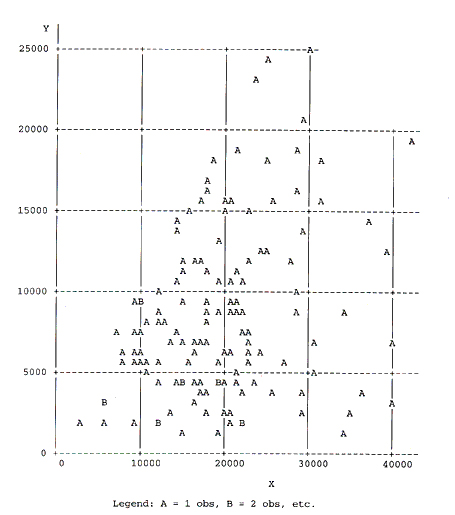Figure 9. Distribution of traffic volumes at signalized four-leg intersections in Washtenaw County, Michigan. X=volume on major, Y=volmum on minor road.

4.1 Smoothing for signalized four-leg intersections

4.2 Analytical models

4.3 Visual comparison of actual data and models

4.4 Stop-controlled intersections

### 4.1 Smoothing for signalized four-leg intersections

Figure 10 shows the results of smoothing with a (4,000 x 4,000) window. The surface shows some waves. The surface obtained with a wider window of (6,000 x 6,000), shown in Figure 11, appears smoother. Using a wider smoothing window resulted in a practically plane surface, which indicates that, over a wide range of traffic volumes, crashes vary essentially linearly with both the major and minor road volumes.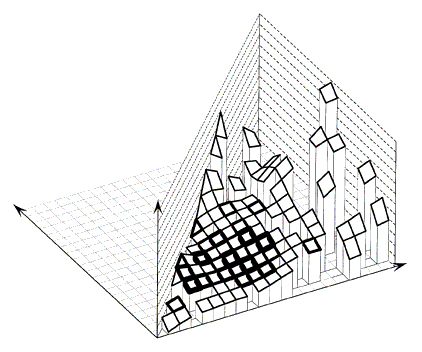Figure 10. Signalized four-leg intersections in Washtenaw County, Michigan. Accident counts smoothed with a 4,000 x 4,000 window.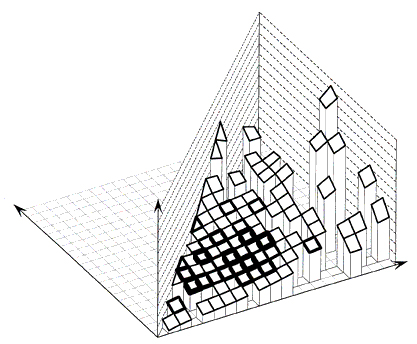Figure 11. Signalized four-leg intersections in Washtenaw County, Michigan. Accident counts smoothed with a 6,000 x 6,000 window.

Top

### 4.2 Analytical models

Because the relation in Figure 11 appeared so smooth and simple, attempts were made to fit analytical models to the data. The simplest model is linear in both volumes. A standard linear regression analysis resulted in

 z=-2+2.07*x+3.78*y (4-1) (7) (0.34) (0.51)

where standard errors are shown in parentheses below the coefficients, and the major volume x and minor volume y are in thousands. The intercept is so small and uncertain that for all practical purposes the function is a plane though the origin, that is, crashes increase independently and proportionally with x and with y. Figure 12 shows this plane.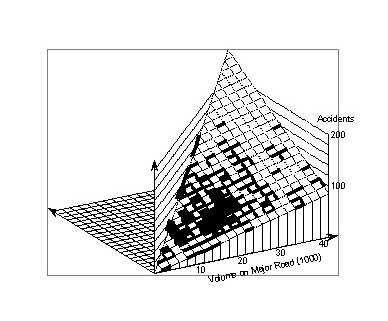Figure 12. Signalized four-leg intersections in Washtenaw County, Michigan. Surface represents the analytical model 4-1.

The simple linear model is not commonly used because it does not reflect an interaction of two traffic streams and is therefore implausible. Most models used are refinements of the model

 z=a*xbyc, (4-2)

which is often fitted to the data in the log-linear form such as,

 log(z) = a+b*log(x)+c*log(y) (4-3)

Analytically, these models are equivalent. Statistically, however, they are different.

Fitting a model of type (4-3) by linear regression to the logarithm of the data gives

 z = 11.6*x0.352*y0.343 (4-4) (9.4)(0.082) (0.052)

It is noteworthy that both exponents agree well within their errors. This means that the function is symmetric in both volumes. Figure 13 shows the corresponding surface. Comparison with Figure 12 shows that the log-linear model "predicts" more crashes than the linear model for low volumes, and fewer crashes for high volumes.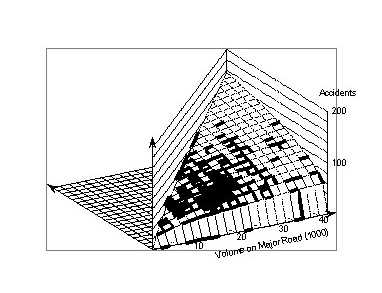Figure 13. Signalized four-leg intersections in Washtenaw County, Michigan. Surface represents the analytical model (4-4).

Fitting a model of type (4-2) using a nonlinear regression routine results in the following:

 z = 3.4*x0.737*y0.405 (4.5) (1.1) (0.101) (0.057)

Here, the exponents of x and y differ well beyond their standard errors. The exponent of x differs very noticeably from that in model (4–4), whereas the exponent of y differs only by about the standard error of their difference, 0.077.

Figure 14 shows the surface representing model (4–5). Comparison with Figure 13 shows that for low volumes the log–linear model "predicts" more crashes, whereas it "predicts" fewer crashes for higher volumes. For actual intersections, the differences are large at the extreme values. In areas without intersections where the model truly "predicts," the differences are dramatic. This raises the question of what is the reason for these discrepancies between models of the same analytical form?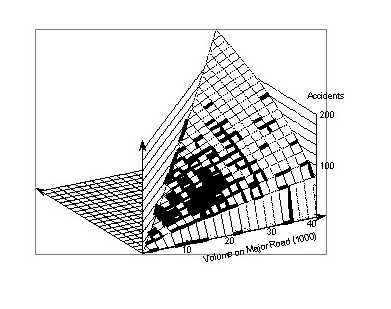Figure 14. Signalized four-leg intersections in Washtenaw County, Michigan. Surface represents the analytical model 4-6.

Assume that the value zi of observation i has a standard error of si. Then, the standard error of ui = log (zi) is t = si/zi. The corresponding weights to be used in the analyses are vi=1/s i 2in the nonlinear regression and wi=1/ti=z12/s12 in the log–linear case. In the simple nonlinear regression, the standard errors of all observations are assumed to be equal. Similarly, in simple log–linear modeling one assumes that the standard errors of the logarithms of the observations are equal. If, in fact, the standard errors of the observations were equal, then the nonlinear regression techniques would provide the correct fit. If simple log–linear models were used, the result could be wrong, because wrong weights were used. The correct weights would have to be proportional to z2, which means observations with high values of z are underweighted. The correct model could be obtained if a weight proportional to z2 was used in the log–linear analysis. The reverse holds if, in fact, the variances of the logarithms of the observations are constant.

How does this relate to the well–known theorem that coefficients of weighted and unweighted models have the same expected values under fairly general conditions? First, though the expected values may be the same, the actually computed values from a finite sample are usually different. Second, the theorem assumes implicitly that the model is "correct," i.e., that it has no systematic errors. In our situation, it is very likely that the arbitrarily selected simple analytical model has some systematic errors.

A practical question then is: What are the "correct" or at least "good" weights to be used in model fitting? The simplest model for crash counts is the Poisson distribution, which assumes that if a crash count z has an expected value m, then its variance is also m. We used this in the fourth model where we fitted a nonlinear model (4–2), but used weights proportional to 1/m. Since the value of m is not known, we proceeded interactively by starting with equal weights and fitting a model. We then used the fitted values of this model as weights for fitting a second model, and so on until the coefficients no longer changed. This procedure is asymptotically equivalent to fitting a maximum likelihood model to the data, using likelihood functions derived from the Poisson distribution.

The result of our iteratively weighted nonlinear modeling is

 z = 7.0*x0.517*y0.309 (4-6) (1.9) (0.091) (0.055)

The coefficients are between those for models (4-4) and 4-5). Figure 15 shows the corresponding surface.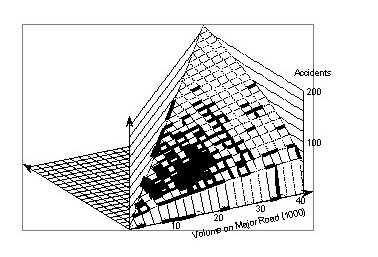Figure 15. Signalized four-leg intersections in Washtenaw County, Michigan. Surface represents the analytical model 4.5.

This model should not be interpreted as being "better" or more "realistic" than the other models. The reason is that the Poisson assumption accounts for only the random component of the residual variance. Even here probably only part of the variation is accounted for because empirical distributions of crash counts tend to be broader than the Poisson – distribution. Very likely there may be systematic–error components, resulting from factors not included in the simplistic models, and from differences between the assumed simple relationship between volumes and crash counts and any "real" relationship. Such potential differences are the reasons why comparing overall measures of goodness of fit, such as R2, or likelihood ratio are not sufficient. They rely heavily on the large number of cases concentrated in certain areas of the x–y plane, and relatively little on the few points outside of these areas. These points, however, may be of greatest interest because the differences between the various model are greatest there. Therefore, in the following section we will visually look at the differences between the models, and between the models and actual data.

Top

### 4.3 Visual comparison of actual data and models

Figures 16 and 17 show cross–sections through the surfaces shown in Figures 11, 12, 13, and 14, at minor volumes of 4,000 vpd and 14,000 vpd, and at major volumes of 16,000 vpd and 30,000 vpd. The locations for these cross–sections were chosen because they touched a wide range of cells that contained intersection data.

Figure 16 shows cross–sections across major volumes. There is a consistent discrepancy between the smoothed curve, approximating the actual values, and the analytical models, except the linear model, at a minor volume of 4,000 vpd. The smoothed curve is convex from below and the models are convex from above. For low values of the major volume, most models predictions are below the actual values; for middle values all are above the actual values; and for the highest values of major volume, all models are below the actual values. None of the models appear clearly superior. At a minor volume of 14,000 vpd, the smoothed curve is convex from below (except for a short piece at high volumes on the major road). All models show nearly straight lines. Again, none of them is consistently better than the others, and none is really "good."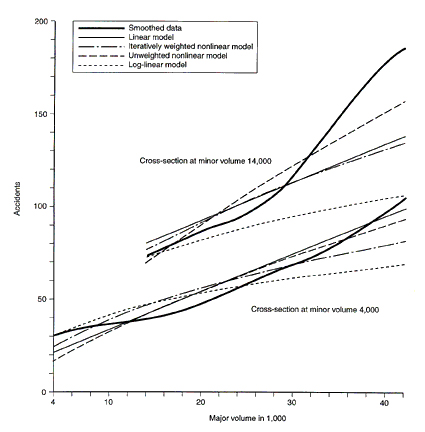Figure 16. Signalized four-leg intersection in Washtenaw County, Michigan. Cross sections through the surfaces in Figures 11, 12, 13, and 14 at mino volumes of 4,000 and 14,000.

Figure 17 shows cross–sections at a major volume of 16,000 vpd across minor volumes. The smoothed relationship is close to a straight line, the linear model is a nearly parallel line, and the nonlinear models are slightly convex from above. The portion of the line below 2,000 vpd should be ignored because there are only 10 intersections with minor volumes between 1,000 vpd and 2,000 vpd, and the curves rely heavily on extrapolation or are forced to zero at zero volume. Aside from this area, the modeled curves are above the smoothed curve nearly everywhere. In this case, the simple unweighted nonlinear model tends to be closest to the smoothed values. At a major volume of 30,000 vpd, the smoothed relationship shows a nearly linear increase with minor volume up to 14,000 vpd. It then levels off and increases above 20,000 vpd. If we again ignore minor volumes below 2,000 vpd, the log–linear model is always much too low, and the unweighted nonlinear model nearly always too high. Overall, the iteratively weighted nonlinear model appears to be the best.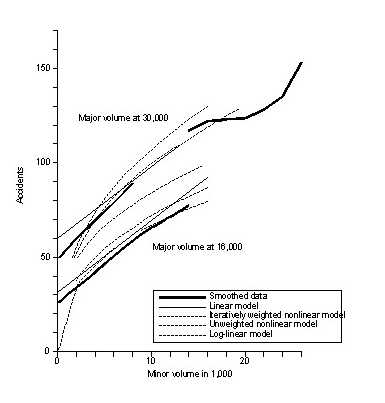Figure 17. Cross-sections at major volume of 16,000.

No clear picture emerges from these comparisons, except that the predictions of crash counts from the simple log–linear model are consistently too low.

Top

### 4.4 Stop–controlled intersections

The range of volume on the major road for stop–controlled intersections was the same as that for signal–controlled intersections. Volumes on the minor road ranged up to the volume of the major road up to 20,000 vpd. At volumes of the major road higher than 20,000 vpd, volumes on the minor road tended to be much lower.

Figure 18 shows the smoothed crash counts using a window of (3,000 x 3,000). Except for a "spike" at very high major, and very low minor volumes, the surface shows no trend. Figure 19 shows the data smoothed with a (6,000 x 6,000) window. Aside from the "spike" that remains, there is no apparent trend of crashes with major volume. At low values of major volume, crashes show a slightly increasing trend with minor volume. This trend disappears at higher values of the major volume.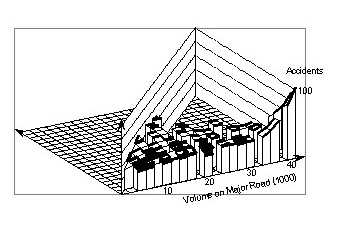Figure 19. Stop–controlled four-leg intersections in Washtenaw County, Michigan. Accident count smoothed with a 6,000 x 6,000 window.

The "spike" is due to a single intersection with 100 crashes, which is not balanced by other intersections with low crash counts. If the effect of this intersection is ignored, the data show such a pattern of variation over a relatively narrow range of crash counts that cannot be represented by the conventional models of the form z = a*xb yc.

Top

FHWA-RD-98-096